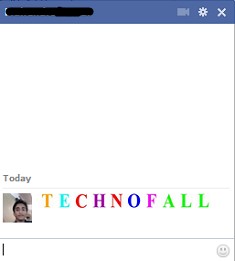# Colorful alphabet and alphabet emoticon for Facebook chat

We all know about different emoticons, smileys which we use during Facebook chat. There are also many toolbars, and softwares available for using emoticons in Facebook. Today i am here with a simple trick that will let you use different alphabet emoticons for Facebook chat like the one used below:Its a very colorful alphabetic message, and your friend will surely message you, asking how did you do that. So for writing these colorful alphabets and alphabet emoticon for Facebook chat, you will have to copy the code with respect to your letter in the chat box and press enter.
Codes from A-Z are provided below.

### CODES for colorful alphabet and alphabet emoticon for Facebook Chat

[] = A
[] = B
[] = C
[] = D
[] = E
[] = F
[] = G
[] = H
[] = I
[] = J
[] = K
[] = L
[] = M
[] = N
[] = O
[] = P
[] = Q
[] = R
[] = S
[] = T
[] = U
[] = V
[] = W
[] = X
[] = Y
[] = Z

NOTE: Give spacing between code of each letter

These were emoticons..
You can write with colorful alphabet also, like i have done in the image below.[] = A
[] = B
[] = C
[] = D
[] = E
[] = F
[] = G
[] = H
[] = I
[] = J
[] = K
[] = L
[] = M
[] = N
[] = O
[] = P
[] = Q
[] = R
[] = S
[] = T
[] = U
[] = V
[] = W
[] = X
[] = Y
[] = Z

NOTE: Give spacing between code of each letter

So copy the codes and let the chat start and surprise your friends with this simple and amazing trick.

Source:HackingUniversity

Stay tunned with us on TechnoFall and get such more tricks.